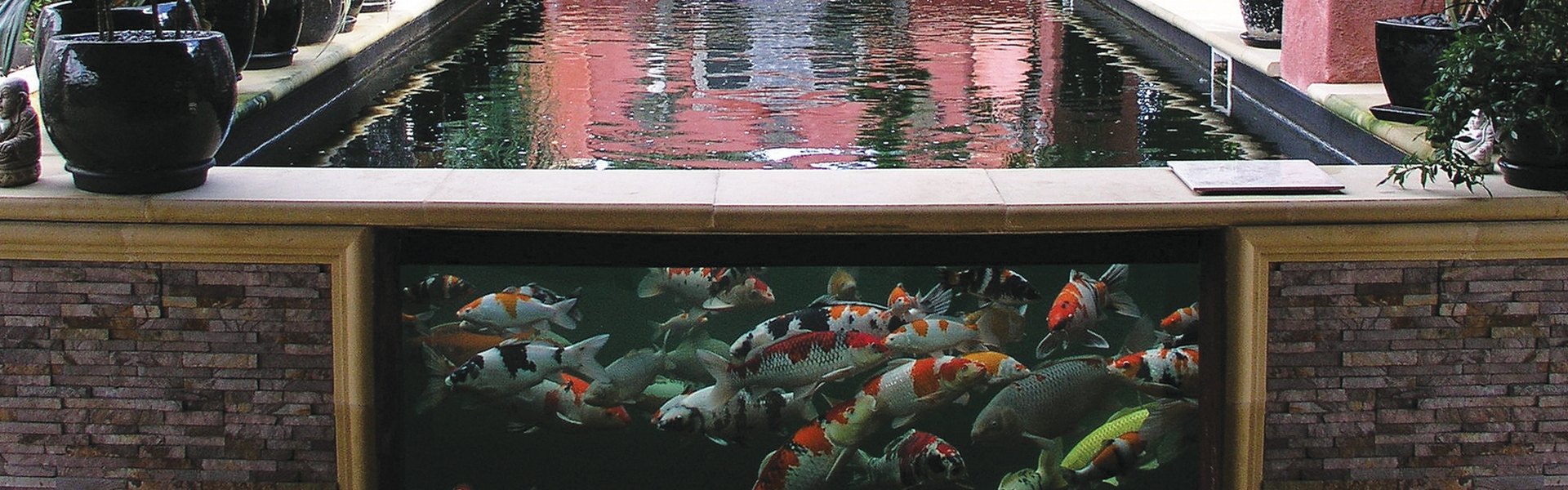## Pond Calculators

Calculate and convert pond sizes with our simple to use calculators

## Pond Volume Calculators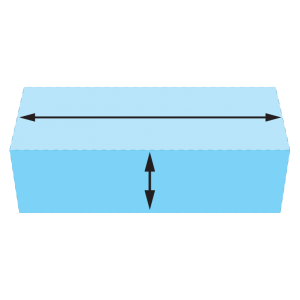Rectangular Pond Calculator
Unit:
Length:
metres/feet
cm/inch
Width:
metres/feet
cm/inch
Depth:
metres/feet
cm/inch
Liner size required
You require a liner: metres long metres wide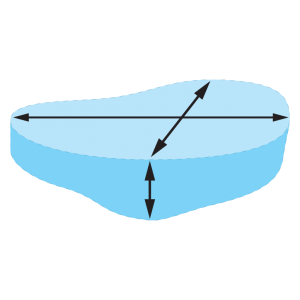Irregular Pond Calculator
Unit:
Av Length:
metre/feet

cm/inch
Av Width:
metre/feet

cm/inch
Av Depth:
metre/feet

cm/inch
Max Length:
metre/feet
cm/inch
Max Width:
metre/feet
cm/inch
Max Depth:
metre/feet
cm/inch
Liner size required
You require a liner: metres long metres wideRound Pond Calculator
Unit:
Av Length:
metres/feet

cm/inch
Av Depth:
metre/feet

cm/inch
Liner size required
You require a liner: metres long metres wide

## Conversion Tools

Use our conversion tools to help you convert metric to imperial and vice versa, to help you select the correct Evolution Aqua products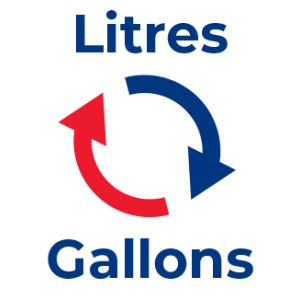Litres & ml to Gallons
litres ml
Converts to

gallons
Gallons to Litres
gallons pints
Converts to

litres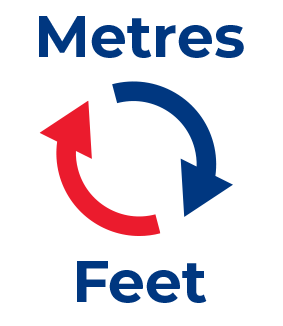Feet & Inches to Metres
feet inches
Converts to

metres
Metres & cm to Feet
metres cm
Converts to

feet